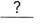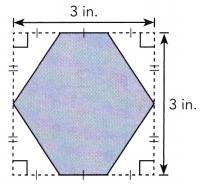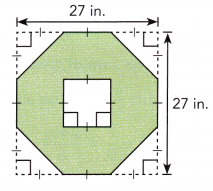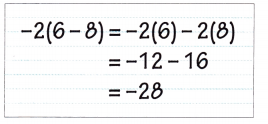# Math in Focus Grade 7 Chapter 2 Lesson 2.4 Answer Key Operations with Integers

Go through the Math in Focus Grade 7 Workbook Answer Key Chapter 2 Lesson 2.4 Operations with Integers to finish your assignments.

## Math in Focus Grade 7 Course 2 A Chapter 2 Lesson 2.4 Answer Key Operations with Integers

### Math in Focus Grade 7 Chapter 2 Lesson 2.4 Guided Practice Answer Key

Evaluate each expression.

Question 1.
14 + 8 – 96
14 + 8 – 9 • 6 = 14 + 8 –Multiply.
= 14 + 8 +Rewrite subtraction as adding the opposite.
= 14 + () + 8 Use the commutative property of addition.
=+ 8 Add.
=Add.
14 + 8 – 9 • 6
= 14 + 8 – 54
= 14 + (-54) + 8
= -40 + 8
= -32
Explanation:
The given expression is 14 + 8 – 9 • 6. Use BODMAS rule.
First we have to perform multiplication operation. Multiply 9 with 6 the product is 54.
We have to use – symbol before 54. Now the expression is 14 + 8 – 54.
Now, use the commutative property of addition the expression is 14 + (-54) + 8.
Add 14 with -54 the sum is -40. The expression is -40 + 8.
Add -40 with 8 the sum is -32.

Question 2.
(-25 – 5) ÷ 6 – 21
(-25 – 5) ÷ 6 – 21 =÷ 6 – 21 Subtract within the parentheses.
=– 21 Divide.
=Subtract.
(-25 – 5) ÷ 6 – 21
= (-30) ÷ 6 – 21
= -5 – 21
= -26
Explanation:
The given expression is (-25 – 5) ÷ 6 – 21. Use BODMAS rule.
First we have to perform operation within the parentheses. Subtract -25 with -5 the difference is -30. Now the expression is  (-30) ÷ 6 – 21.
Secondly we have to perform division operation. Divide -30 by 6 the quotient is -5.
The expression is -5 -21. Subtract -5 with – 21 the result is -26.

Question 3.
-14 – (3 + 3) • 2
-14 – (3 + 3) • 2
= -14 – (6) • 2
= -14 – 12
= – 26
Explanation:
The given expression is -14 – (3 + 3) • 2. Use BODMAS rule.
First we have to perform operation within the parentheses. Add 3 with 3 the sum is 6. Now the expression is  -14 -(6) • 2
Second we have to perform multiplication operation. Multiply 6 with 2 the product is 12. Now the expression is -14 -12.
Third we have to perform subtraction operation. Subtract -14 with -12 the result is -26.

Solve.

Question 4.
Joseph drew a hexagon on a 3-inch square piece of paper. He cut four identical right triangles from the four corners of the paper. The height of each triangle is $$\frac{1}{2}$$ the length of the paper. The base of each triangle is $$\frac{1}{3}$$ the length of the paper. What is the area of the paper that remained after these triangles are cut off?Height of a triangle:Base of a triangle:Area of remaining paper:
Area of original paper – Area of four cut-off triangles
= 3 • 3 – 4 • ($$\frac{1}{2}$$ •) Write an expression.
=Multiply.
=in2 Subtract.
The area of the remaining paper issquare inches.
Length of the paper = 3 inches
Height of a triangle: 1/2 • length of the paper = 1/2 • 3 = 3/2
Base of a triangle: 1/3 • length of the paper = 1/3 • 3 = 1
Area of four cut-off triangles = 4 • (1/2 • base • height)
Area of remaining paper = Area of original paper – Area of four cut-off triangles
= 3 • 3 – 4 • (1/2 • 1 • 3/2)
= 9 – 4 •(3/4)
= 9 – 3
= 6 in2
The area of the remaining paper is 6 square inches.

### Math in Focus Course 2A Practice 2.4 Answer Key

Evaluate each expression.

Question 1.
-3 • 5 + 7
-3 • 5 + 7
= – 15 + 7
= -8
Explanation:
The given expression is -3 • 5 + 7. Use BODMAS rule.
First multiply -3 with 5 the product is -15. Now the expression is -15 + 7.
Next perform addition operation. Add -15 with 7 the sum is -8.

Question 2.
50 ÷ (-5) + (-4)
50 ÷ (-5) + (-4)
= 50 ÷ -5 – 4
= -10 – 4
= -14
Explanation:
The given expression is 50 ÷ (-5) + (-4). Use BODMAS rule.
First perform operations within the parentheses. Now the expression is 50 ÷ – 5 – 4.
Next perform division operation. Divide 50 by -5 the quotient is -10. Now the expression is -10 -4.
Subtract -10 with -4 the result is -14.

Question 3.
4 • (-6) + (-3) • 5
4 • (-6) + (-3) • 5
= 4 • -6  – 3 • 5
= -24 – 15
= -39
Explanation:
The given expression is 4 • (-6) + (-3) • 5. Use BODMAS rule.
First perform operations within the parentheses. Now the expression is 4 • -6  – 3 • 5.
Next perform multiplication operation. Multiply 4 with -6 the product is -24. Multiply -3 with 5 the product is -15. Now the expression is -24 – 15.
Subtract -24 with -15 the result is -39.

Question 4.
11 – 2 • 8 – (-9)
= 11 – 2 • 8 – (-9)
= 11 – 2 • 8 + 9
= 11 – 16 + 9
= 20 – 16
= 4
Explanation:
The given expression is 11 – 2 • 8 – (-9). Use BODMAS rule.
First perform operations within the parentheses. Now the expression is 11 – 2 • 8 + 9.
Next perform multiplication operation. Multiply 2 with 8 the product is 16. Now the expression is 11 – 16 + 9.
Add 11 with 9 the sum is 20. The expression is 20 -16.
Subtract 16 from 20 the result is 4.

Question 5.
-64 ÷ 4. 5 – 37
-64 ÷ 4. 5 – 37
= -16. 5 – 37
= – 80 – 37
= – 117
Explanation:
The given expression is-64 ÷ 4. 5 – 37. Use BODMAS rule.
First perform division operation. Divide 64 by 4 the quotient is 16. Now the expression is -16. 5 – 37.
Next perform multiplication operation. Multiply 16 with 5 the product is 80. Now the expression is -80 – 37.
subtract -80 with -37 the result is -117.

Question 6.
-28 – 350 ÷ 7 + 8
-28 – 350 ÷ 7 + 8
= -28 – 50 + 8
= -28 -42
= – 70
Explanation:
The given expression is -28 – 350 ÷ 7 + 8. Use BODMAS rule.
First perform division operation. Divide 350 by 7 the quotient is 50. Now the expression is -28 – 50 + 8.
Next perform addition operation. Add -50 with 8 the sum is -42. Now the expression is -28 -42.
subtract -28 with -42 the result is -70.

Question 7.
1oo – (8 – 15) • 9
1oo – (8 – 15) • 9
= 100 – (-7) • 9
= 100 + 63
= 163
Explanation:
The given expression is 1oo – (8 – 15) • 9. Use BODMAS rule.
First perform operations within the parentheses. Subtract 15 from 8 the difference is -7. Now the expression is 100 – (-7) • 9.
Next perform multiplication operation. Multiply -7 with 9 the product is -63. Now the expression is 100 + 63.
Add 100 with 63 the sum is 163.

Question 8.
70 ÷ (-4 – 3) + 60
70 ÷ (-4 – 3) + 60
= 70 ÷ (-7) + 60
= – 10 + 60
= 50
Explanation:
The given expression is 70 ÷ (-4 – 3) + 60. Use BODMAS rule.
First perform operations within the parentheses. Subtract -4 with -3 the result is -7. Now the expression is 70 ÷ (-7) + 60.
Next perform division operation. Divide 70 by – 7 the quotient is -10. Now the expression is -10 + 60.
Add -10 with 60 the result is 50.

Question 9.
(4 + 2) • 3 – 8 • (2 + 3)
(4 + 2) • 3 – 8 • (2 + 3)
= 6 • 3 – 8 • 5
= 18 – 40
= -22
Explanation:
The given expression is (4 + 2) • 3 – 8 • (2 + 3). Use BODMAS rule.
First perform operations within the parentheses. Add 4 with 2 the sum is 6. Add 2 with 3 the sum is 5. Now the expression is 6 • 3 – 8 • 5.
Next perform multiplication operation. Multiply 6 with 3 the product is 18. Multiply 8 with 5 the product is 40. Now the expression is 18 – 40.
Subtract 40 from 18 the difference is -22.

Question 10.
70 ÷ (-4 – 3) + 60
= 70 ÷ (-7) + 60
= – 10 + 60
= 50
Explanation:
The given expression is 70 ÷ (-4 – 3) + 60. Use BODMAS rule.
First perform operations within the parentheses. Subtract -4 with -3 the result is -7. Now the expression is 70 ÷ (-7) + 60.
Next perform division operation. Divide 70 by – 7 the quotient is -10. Now the expression is -10 + 60.
Add -10 with 60 the result is 50.

Question 11.
15 ÷ (4 + 1) – 8.3
15 ÷ (4 + 1) – 8 • 3
= 15 ÷ 5 – 8 • 3
= 3 – 8 • 3
= 3 – 24
= -21
Explanation:
The given expression is 15 ÷ (4 + 1) – 8 • 3. Use BODMAS rule.
First perform operations within the parentheses. Add 4 with 1 the sum is 5. Now the expression is 15 ÷ 5 – 8 • 3.
Next perform division operation. Divide 15 by 5 the quotient is 3. Now the expression is 3 – 8 • 3.
Perform multiplication operation. Multiply 8 with 3 the product is 24. Now the expression is 3 – 24.
Subtract 24 from 3 the difference is -21.

Question 12.
24 ÷ 4 – (-13 + 3) • 2
24 ÷ 4 – (-13 + 3) • 2
= 24 ÷ 4 + 10 • 2
= 6 + 10 • 2
= 6 + 20
= 26
Explanation:
The given expression is 24 ÷ 4 – (-13 + 3) • 2. Use BODMAS rule.
First perform operations within the parentheses. Add 3 with -13 the sum is -10. Now the expression is 24 ÷ 4 + 10 • 2.
Next perform division operation. Divide 24 by 4 the quotient is 6. Now the expression is 6 + 10 • 2.
Perform multiplication operation. Multiply 10 with 2 the product is 20. Now the expression is 6 + 20.
Add 6 with 20 the sum is 26.

Question 13.
-12 + 50 ÷ (-2 – 3) + 72 ÷ (4 + 2)
-12 + 50 ÷ (-2 – 3) + 72 ÷ (4 + 2)
= -12 + 50 ÷ (-5) + 72 ÷  6
= -12 – 10 + 12
= -12 + 2
= -10
Explanation:
The given expression is -12 + 50 ÷ (-2 – 3) + 72 ÷ (4 + 2). Use BODMAS rule.
First perform operations within the parentheses. Add -2 with -3 the sum is -5.  Add 4 with 2 the sum is 6. Now the expression is-12 + 50 ÷ (-5) + 72 ÷  6.
Next perform division operation. Divide 50 by -5 the quotient is -10. Divide 72 by 6 the quotient is 12. Now the expression is -12 – 10 + 12.
Add -10 with 12 the sum is 2. Now the expression is -12 + 2.
Add -12 with 2 the sum is -10.

Question 14.
180 ÷ (4 + 16) – 8 • 3 + 7 • (2 + 3)
180 ÷ (4 + 16) – 8 • 3 + 7 • (2 + 3)
= 180 ÷ 20 – 8 • 3 + 7 • 5
= 9 –  8 • 3 + 7 • 5
= 9 – 24 + 35
= 9 + 11
= 20
Explanation:
The given expression is 180 ÷ (4 + 16) – 8 • 3 + 7 • (2 + 3). Use BODMAS rule.
First perform operations within the parentheses. Add 4 with 16 the sum is 20. Add 2 with 3 the sum is 5. Now the expression is 180 ÷ 20 – 8 • 3 + 7 • 5.
Next perform division operation. Divide 180 by 20 the quotient is 9. Now the expression is9 –  8 • 3 + 7 • 5.
Perform multiplication operation. Multiply 8 with 3 the product is 24. Multiply 7 with 5 the product is 35. Now the expression is 9 – 24 + 35.
Add -24 with 35 the sum is 11.
Add 9 with 11 the sum is 20.

Question 15.
Emily made a sketch of an octagonal window on a 27-inch square piece of paper. First she cut four identical isosceles triangles from the corners of the paper. Then she cut a square from the center of the octagon. Each leg of a cut-off triangle is $$\frac{1}{3}$$ the length of the paper. The side length of the cut-out square is also $$\frac{1}{3}$$ the length of the paper. What ¡s the area of the sketch after she removed the triangles and the square?Length of the paper = 27-inch
Area of square piece of paper = side • side = 27 in • 27 in = 729 square inches
Each leg of a cut-off triangle = 1/3 • length of the paper = 1/3 • 27 =9 inches
Side length of a cut-out square = 1/3 • length of the paper = 1/3 • 27 = 9 inches
Area of a square = side x side
Area of four cut-off triangles = 4 • (1/2 • base • height)
From the above image we can observe both base and height are same.
Area of octagonal window = Area of a square piece of paper – Area of four cut-off triangles – Area of a square
= 729 – 4 • (1/2 • 9 • 9) –  9 • 9
= 729 – 4 • (81/2) – 81
= 729 – 2 • 81 – 81
= 729 – 162 – 81
= 486 square inches
The area of the sketch after she removed the triangles and the square is 486 square inches.

Question 16.
Math Journal Suppose that Lydia shows you some of her homework:Lydia made a common error when she used the distributive property to evaluate the expression -2(6 – 8). Evaluate the expression using the order of operations. Then explain how Lydia can correctly use the distributive property to evaluate the expression.
Distributive property = A ( B+ C) = AB + AC
Use distributive property to evaluate the given expression -2 (6 – 8).
-2 (6 – 8) = -2 • 6 + (-2) • (-8)
= -12 + 16
= 4
– 2 (6 – 8) = 4

Question 17.
Sylvia took three turns in a video game. She scored -120 points during her first turn, 320 points during her second turn, and -80 points during her third turn. What was her average score for the three turns?
Sylvia scored -120 points during her first turn.
Sylvia scored 320 points during her second turn.
Sylvia scored -80 points during her third turn.
Average = (-120 + 320 -80)/ 3 = 120/3 = 40
Average score for the three turns is 40 points.

Question 18.
Math Journal Benjamin wrote: -20 + 4 • 2 + 7 – 35 = -19. Where can he place the parentheses so that the equation will be a true statement?